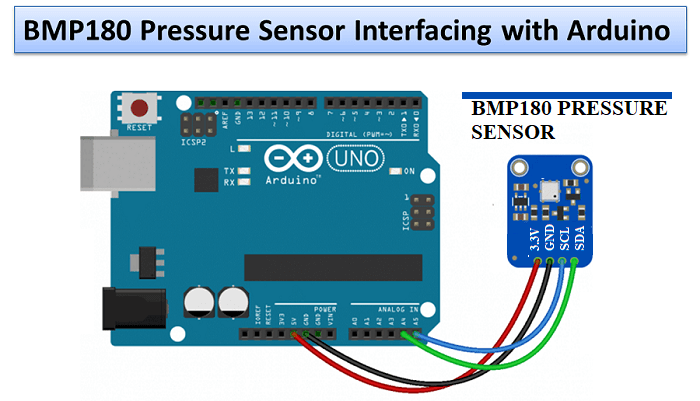# Pressure sensor BMP180 with Arduino

What is Pressure, Atmospheric or Barometric Pressure? How to interface pressure sensor with arduino?

To measure atmospheric pressure, we use pressure sensor BMP180. Pressure is a force that acts on per unit area.

When one pound force presses one square inch area then this pressure is known as one psi (pound per square inch).

The SI unit of pressure is Pascal (Pa), force of one newton per square meter (N/m2) which is known as 1 Pascal (Pa).

## WHAT IS BAROMETRIC or ATMOSPHERIC PRESSURE?

Atmospheric pressure is simply the force, whose effect exists on all the stuff we are surrounded by. Actually the weight of gases present in the atmosphere pressing down on the earth creates the atmospheric pressure.

Think a column of air going up from the surface of the earth to the top of the atmosphere. Air has mass, so the gravity tries to attract it, which causes the air column’s weight to exert pressure on the earth.

The pressure produced by a 1 Inch × 1 Inch column of air reaching to the top of the atmosphere is known as one atmosphere (atm) of pressure. This column of air weighs 14.7 pounds, that’s why one atm equals to 14.7 pounds per square inch (psi).

## PRESSURE UNIT CONVERSION TABLE

 Unit 1 hPa  (hectoPascals) = Pascal 100 Pa Newton per square meter 100 N/m Atmosphere 0.000986923 atm Bar 0.001 bar Millibar 1 mbar Millimeters Mercury 0.750063755 mmHg Torr 0.750061683 torr Pounds per square inch 0.014503774 psi

## HOW THE BMP180 WORKS

The BMP180 is a piezoresistive sensor used in measuring pressure. These are commonly made up of semiconductor material. The piezoresistive effect is a change in electrical resistance of metal when mechanical force is applied, like atmospheric pressure, pushing with hands etc.

With BMP180 we can measure both important parameters required for finding pressure, those are temperature and pressure. See the pressure formula given below

Ph = P0e-mgh/kT

• m = mass of one air molecule
• g = acceleration due to gravity
• k = Boltzmann’s constant (ideal gas constant divided by Avogadro’s number)
• T = temperature
• The sea level pressure (p0)
• Pressure at height h (ph)

As we know, the density of gases can change with change in temperature. As temperature rises air molecules will expand themselves and rise and when the temperature cools down then molecules will be denser and contract.

For example

• The same process happens at the time of water vapour & cloud formation (water recycle)
• In hot air balloonThe BMP180 finds the amount of uncompensated temperature (UT) and uncompensated pressure (UP). The calculation of temperature is taken first, and then a pressure test follows.

This flow chart details the steps taken by the sensor when a calculation is carried out:The BMP180 has an EEPROM of 176 bits and includes 11 separate calibration coefficients unique to each sensor. These are used together with the UP and UT for measuring the true barometric pressure and temperature.

## BMP180 PRESSURE SENSOR PINOUT DIAGRAM## CONNECTING THE BMP180 TO THE ARDUINO

The BMP180 interacts over I2C to Arduino. Different Arduino board I2C pins (SDA and SCL) position and sequence differs from each other. Check the table below to find some common Arduino boards with the I2C pins:

 Arduino SDA Pin SCL Pin UNO / NANO / MINI A4 A5 MEGA / DUE 2 3 MICRO / LEONARDO 20 21

The Bosch BMP180 operates on 3.3V (recommended), but many breakout boards are fitted with a voltage regulator and an I2C level shifter to control it either with 3.3V or 5V.

### PRESSURE SENSOR WITH ARDUINO CIRCUIT DIAGRAM## PRESSURE SENSOR WITH ARDUINO PROGRAMMING CODE

```/* Do connection as follows for Arduino uno: VCC to +5V, Gnd to Gnd, SDA to A4, SCL to A5
for other board connection, Click Tutorial link:  https://pijaeducation.com/arduino/sensor/pressure-sensor-with-arduino/

#include <Wire.h>
#include <SFE_BMP180.h>
SFE_BMP180 bmp180;

double T, P;
double p, t;
bool success = false;

void setup() {
Serial.begin(9600);
bool success = bmp180.begin();
if (success) {
Serial.println("BMP180 init success");
}
}

void loop() {

// startTemperature(), Will return delay in ms to wait, or 0 if I2C error.
int statusST = bmp180.startTemperature();
//  Serial.print("statusST : ");
//  Serial.println(statusST);

if (statusST != 0) {
delay(1000);
// getTemperature(T), Returns 1 if successful, 0 if I2C error.zz
// T: external variable to hold result.
int statusGT = bmp180.getTemperature(T);
//    Serial.print("statusGT : ");
//    Serial.println(statusGT);

if (statusGT != 0) {
// startPressure(Oversampling), Oversampling: 0 to 3, higher numbers are slower, higher-res outputs
// 0 for delay 5, 1 for delay 8, 2 for delay 14 and 3 for delay 26, default delay 5
int statusSP = bmp180.startPressure(3);
//      Serial.print("statusSP : ");
//      Serial.println(statusSP);

if (statusSP != 0) {
delay(statusSP);
// P: external variable to hold pressure.
// T: previously-calculated temperature.
// Returns 1 for success, 0 for I2C error.

int statusGP = bmp180.getPressure(P, T);
//        Serial.print("statusGT : ");
//        Serial.println(statusGT);

if (statusGP != 0) {
// uncomment this function, if you want to print, either pressure or temperature value get change by 1.0
// onChangeBy1();

Serial.print("Pressure: ");
Serial.print(P);
Serial.println(" hPa");

Serial.print("Temperature: ");
Serial.print(T);
Serial.println(" C");
}
}
}
}
}

void onChangeBy1() {
if ((P - p) >= 1 || (p - P) >= 1) {
Serial.println("CHANGE DETECTED PRESSURE: ");
p = P;
t = T;
Serial.print("Pressure :"); Serial.print(p); Serial.print(" hPa");
Serial.print(", Temperature :"); Serial.print(t); Serial.println(" C");
}
if ((T - t) >= 1 || (t - T) >= 1) {
Serial.println("CHANGE DETECTED TEMPERATURE: ");
p = P;
t = T;
Serial.print("Pressure :"); Serial.print(p); Serial.println(" hPa");
Serial.print(", Temperature :"); Serial.print(t); Serial.println(" C");
}

}```

### OUTPUTMOTOR WITH ARDUINO

Subscribe
Notify of